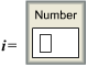# Problem: For an aqueous solution of HF, determine the van't Hoff factor assuming... 0% ionization                                     100% ionization                                    A solution is made by dissolving 0.0300 mol of HF in 1.00 kg of water. The solution was found to freeze at -0.0644 °C. Calculate the value of i and estimate the percent ionization of HF in this solution. so HF is a) 0% ionizedb) between 0% and 50% ionizedc) 50% ionizedd) between 50% and 100% ionizede) 100% ionizedin this solution.

###### FREE Expert Solution

$\overline{){{\mathbf{\Delta T}}}_{{\mathbf{f}}}{\mathbf{=}}{{\mathbf{imK}}}_{{\mathbf{f}}}}$

i = 1 → non-electrolyte or compounds that do not ionize

i = # of ions produce → electrolytes or compounds that 100% ionize###### Problem Details

For an aqueous solution of HF, determine the van't Hoff factor assuming...

0% ionization                                     100% ionizationA solution is made by dissolving 0.0300 mol of HF in 1.00 kg of water. The solution was found to freeze at -0.0644 °C. Calculate the value of i and estimate the percent ionization of HF in this solution.so HF is

a) 0% ionized

b) between 0% and 50% ionized

c) 50% ionized

d) between 50% and 100% ionized

e) 100% ionized

in this solution.